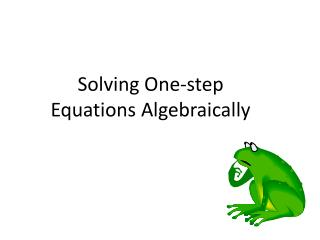# Solving One-step Equations Algebraically - PowerPoint PPT PresentationDownload PresentationSolving One-step Equations Algebraically

Solving One-step Equations AlgebraicallyDownload Presentation## Solving One-step Equations Algebraically

- - - - - - - - - - - - - - - - - - - - - - - - - - - E N D - - - - - - - - - - - - - - - - - - - - - - - - - - -
##### Presentation Transcript

1. Solving One-step Equations Algebraically

2. Today, we will connect modeling equations with algebra tiles to solving equations algebraically. • Our goal and rule for solving equations, however, have not changed. The goal of solving equations: -To get one x alone on one side of the equation. The rule for solving equations: -Do the same thing to both sides of the equation.

3. Let’s start with an addition equation… x + 2 = 7 With algebra tiles Algebraically x + 2 = 7 x + 2 = 7 • To get x alone, take 2 units from each side. • In math, “take away” means to subtract. - 2 - 2 x = 5 X = 5 Think opposite operations: To undo “x plus 2,” we subtracted 2.

4. Let’s try a multiplication equation… 3x = 15 With algebra tiles Algebraically 3x = 15 3x = 15 • To get x alone, split both sides into 3 groups. • In math, “splitting into groups” means to divide. 3 3 x = 5 X = 5 Think opposite operations: To undo “3 times x,” we divided by 3.

5. Now for a subtraction equation… x – 3 = 8 With algebra tiles Algebraically x – 3 = 8 x + -3 = 8 x – 3 = 8 • To get x alone, add 3 units to both sides. • In math, “adding 3 ” means + 3. + 3 + 3 x = 11 X = 11 Think opposite operations: To undo “x minus 3,” we added 3.

6. We did not model a division equation. Based on the previous examples, can you guess what will happen? How will we “undo” division? Algebraically (5) (5) We will use multiplication to “undo” division. In this case, we will multiply both sides by 5. x = 20 Think opposite operations: To undo “x divided by 5,” we multiplied by 5.